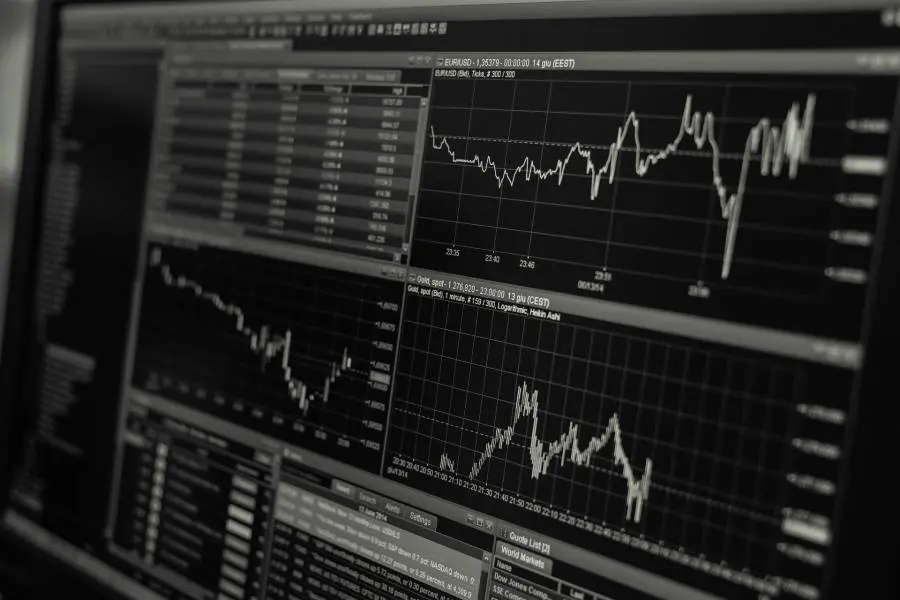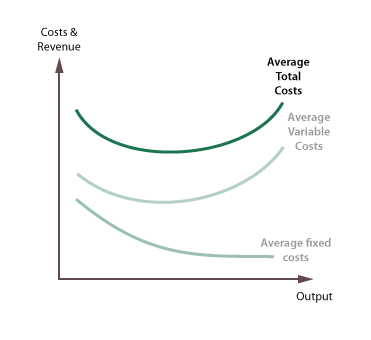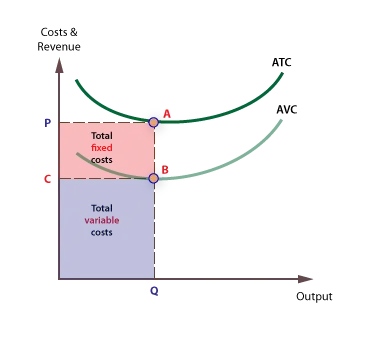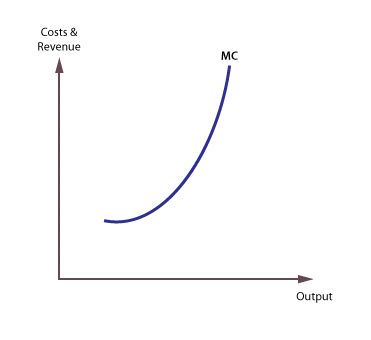# Costs

## Costs of production

### Fixed and variable costs

Fixed costs are those that do not vary with output and typically include rents, insurance, depreciation, set-up costs, and normal profit. They are also called overheads.

Variable costs are costs that do vary with output, and they are also called direct costs. Examples of typical variable costs include fuel, raw materials, and some labour costs.

### Try a quiz on costs

#### An example

Consider the following hypothetical example of a boat building firm. The total fixed costs, TFC, include premises, machinery and equipment needed to construct boats, and are £100,000, irrespective of how many boats are produced. Total variable costs (TVC) will increase as output increases.

 OUTPUT TOTAL FIXED COST TOTAL VARIABLE COST TOTAL COST 1 100 50 150 2 100 80 180 3 100 100 200 4 100 110 210 5 100 150 250 6 100 220 320 7 100 350 450 8 100 640 740

Plotting this gives us Total Cost, Total Variable Cost, and Total Fixed Cost.### Total fixed costs

Given that total fixed costs (TFC) are constant as output increases, the curve is a horizontal line on the cost graph.

### Total variable costs

The total variable cost (TVC) curve slopes up at an accelerating rate, reflecting the law of diminishing marginal returns.

### Total costs

The total cost (TC) curve is found by adding total fixed and total variable costs. Its position reflects the amount of fixed costs, and its gradient reflects variable costs.

### Average fixed costs

Average fixed costs are found by dividing total fixed costs by output. As fixed cost is divided by an increasing output, average fixed costs will continue to fall.

 OUTPUT TOTAL FIXED COST (£000) AVERAGE FIXED COST (£000) 1 100 100 2 100 50 3 100 33.3 4 100 25 5 100 20 6 100 16.6 7 100 14.3 8 100 12.5The average fixed cost (AFC) curve will slope down continuously, from left to right.

### Average variable costs

Average variable costs are found by dividing total fixed variable costs by output.

 OUTPUT TOTAL VARIABLE COST (£000) AVERAGE VARIABLE COST (£000) 1 50 50 2 80 40 3 100 33.3 4 110 27.5 5 150 30 6 220 36.7 7 350 50 8 640 80The average variable cost (AVC) curve will at first slope down from left to right, then reach a minimum point, and rise again.

AVC is ‘U’ shaped because of the principle of variable Proportions, which explains the three phases of the curve:

1. Increasing returns to the variable factors, which cause average costs to fall, followed by:
2. Constant returns, followed by:
3. Diminishing returns, which cause costs to rise.

### Average total cost

Average total cost (ATC) is also called average cost or unit cost. Average total costs are a key cost in the theory of the firm because they indicate how efficiently scarce resources are being used. Average variable costs are found by dividing total fixed variable costs by output.

 OUTPUT AVERAGE FIXED COST (£000) AVERAGE VARIABLE COST (£000) AVERAGE TOTAL COSTS (£000) 1 100 50 150 2 50 40 90 3 33.3 33.3 67 4 25 27.5 52.5 5 20 30 50 6 16.6 36.7 53.3 7 14.3 50 64.3 8 12.5 80 92.5Average total cost (ATC) can be found by adding average fixed costs (AFC) and average variable costs (AVC). The ATC curve is also ‘U’ shaped because it takes its shape from the AVC curve, with the upturn reflecting the onset of diminishing returns to the variable factor.

### Areas for total costs

Total Fixed costs and Total Variable costs are the respective areas under the Average Fixed and Average Variable cost curves.### Marginal costs

Marginal cost is the cost of producing one extra unit of output.  It can be found by calculating the change in total cost when output is increased by one unit.

 OUTPUT TOTAL COST MARGINAL COST 1 150 2 180 30 3 200 20 4 210 10 5 250 40 6 320 70 7 450 130 8 740 290

It is important to note that marginal cost is derived solely from variable costs, and not fixed costs.

The marginal cost curve falls briefly at first, then rises. Marginal costs are derived from variable costs and are subject to the principle of variable proportions.### The significance of marginal cost

The marginal cost curve is significant in the theory of the firm for two reasons:

1. It is the leading cost curve, because changes in total and average costs are derived from changes in marginal cost.
2. The lowest price a firm is prepared to supply at is the price that just covers marginal cost.

### ATC and MC

Average total cost and marginal cost are connected because they are derived from the same basic numerical cost data.  The general rules governing the relationship are:

1. Marginal cost will always cut average total cost from below.
2. When marginal cost is below average total cost, average total cost will be falling, and when marginal cost is above average total cost, average total cost will be rising.
3. A firm is most productively efficient at the lowest average total cost, which is also where average total cost (ATC) = marginal cost (MC).

### Total costs and marginal costs

Marginal costs are derived exclusively from variable costs, and are unaffected by changes in fixed costs. The MC curve is the gradient of the TC curve, and the positive gradient of the total cost curve only exists because of a positive variable cost. This is shown below:### Sunk costs

Sunk costs are those that cannot be recovered if a firm goes out of business. Examples of sunk costs include spending on advertising and marketing, specialist machines that have no scrap value, and stocks which cannot be sold off.

Sunk costs are a considerable barrier to entry and exit.